Get instant live expert help with Excel or Google Sheets“My Excelchat expert helped me in less than 20 minutes, saving me what would have been 5 hours of work!”

#### Post your problem and you’ll get Expert help in seconds.

Your message must be at least 40 characters
Our professional Expert are available now. Your privacy is guaranteed.

# How to Count Unique Text Values in a Range in Excel

We can count unique text values in a range by using a combination of FREQUENCY, MATCH, ROW and SUMPRODUCT functions. We can also achieve the same result with the COUNTIF function. The steps below will walk through the process.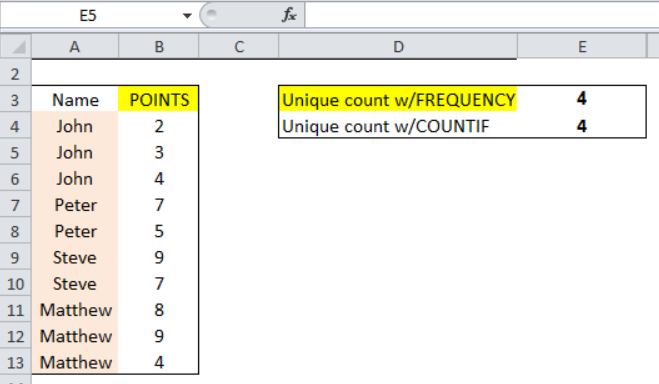Figure 1: Result of Count of Unique Text Values in a Range

## General formula

`=SUMPRODUCT(--(FREQUENCY(MATCH(txt,txt,0),ROW(txt)-ROW(txt.firstcell+1)>0))`

”txt” is the range containing the text or names

## Formula

`=SUMPRODUCT(--(FREQUENCY(MATCH(A4:A13,A4:A13,0),ROW(A4:A13)-ROW(A4)+1)>0))`

## Setting up the Data

We will set up the table as shown in figure 2 with hypothetical players who have scored some points. Now, we have players whose names have appeared more than once. Our intention is to count the number of unique names that appear.

• We will input the names in range A4:A13
• The points will be placed in Column B
• Cell E3 and Cell E4 will contain the results by applying the FREQUENCY and COUNTIF functions respectively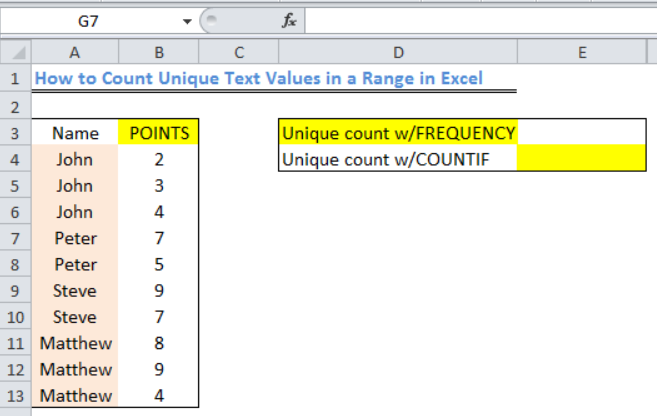Figure 2: Setting up the Data

Applying the Frequency Function

• We will insert the formula below into Cell E3

`=SUMPRODUCT(--(FREQUENCY(MATCH(A4:A13,A4:A13,0),ROW(A4:A13)-ROW(A4)+1)>0))`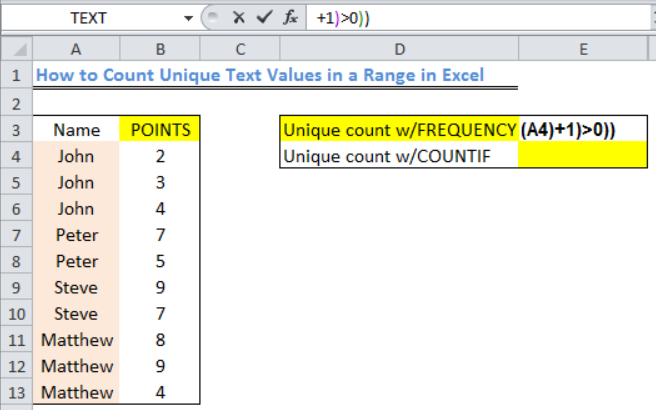Figure 3: How to Count Unique Text Values in a Range

• We will press the enter key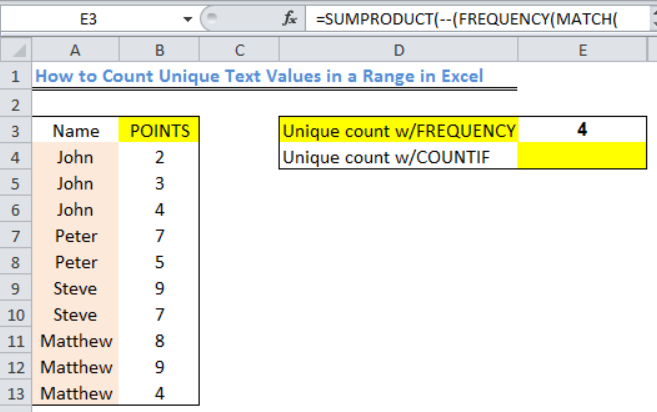Figure 4: Result of Unique Text Values in the Range

## Applying the COUNTIF Function

• We will insert the formula below into Cell E4

`=SUMPRODUCT(1/COUNTIF(A4:A13,A4:A13))`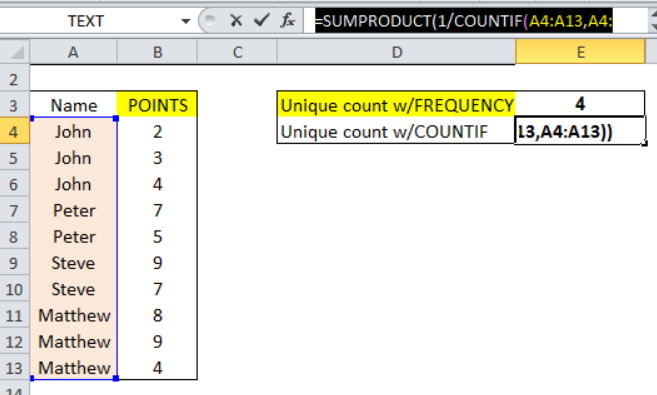Figure 5: How to Count Unique Text Values in a Range with the COUNTIF function

• We will press the enter key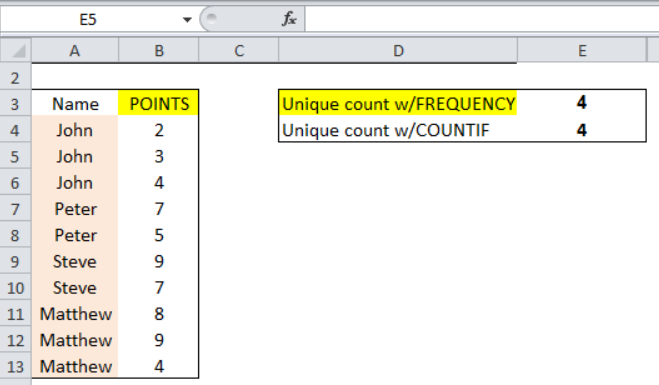Figure 6: Result of Count of Unique Text Values in a Range

## Explanation

`=SUMPRODUCT(--(FREQUENCY(MATCH(A4:A13,A4:A13,0),ROW(A4:A13)-ROW(A4)+1)>0))`

• MATCH Function

This function gets the position of each name in the range. MATCH only returns the position of the first match in the range, as a result, any name that appears more than once will bear the same number so that the returned array of this example looks like this:

`{1;1;1;4;4;6;6;8;8;8}`

• ROW Function

`ROW(A4:A13)-ROW(A4)+1`

The argument above is used to build a straight and sequential array. This is achieved by using the row number of each item in the range and the first item in the range. The returned array will look like this:

`{1;2;3;4;5;6;7;8;9;10}`

• FREQUENCY Function

The FREQUENCY function does not work with non-numeric data; hence, the bulk of the formula will convert the data into numeric data that FREQUENCY works with.

The FREQUENCY function uses the array supplied by MATCH and that supplied by ROW.

The FREQUENCY function will return an array of values that shows the count for every value that is present in the array. FREQUENCY will return zero for any number that repeats itself in the array. The resultant array will look like this:

`{3;0;0;2;0;2;0;3;0;0;0}`

• SUMPRODUCT Function

The returned array from FREQUENCY is evaluated by TRUE or FALSE to know if the numbers are greater than zero. Afterward, TRUE values are returned as 1 and FALSE as 0. The resultant array in SUMPRODUCT will look like this:

`{1;0;0;1;0;1;0;1;0;0;0}`

SUMPRODUCT then sums the values and the result is 4 as shown.

## Instant Connection to an Expert through our Excelchat Service

Most of the time, the problem you will need to solve will be more complex than a simple application of a formula or function. If you want to save hours of research and frustration, try our live Excelchat service! Our Excel Experts are available 24/7 to answer any Excel question you may have. We guarantee a connection within 30 seconds and a customized solution within 20 minutes.

### Did this post not answer your question? Get a solution from connecting with the expert.Another blog reader asked this question today on Excelchat:
Solution examplesI need a formula to compare the data in two columns and then export the mismatched data in the 3rd column
Solved by S. Q. in 20 minsi have a table with Dates, First names , Last names and i want to count how many times a name occurs, but if a name occurs more than once on a particular date i need to only count it once. this is on excel and not google sheets
Solved by F. H. in 31 minsUse the Vlookup Function to complete the "employee" column of table 2. Use "job Id" from table 2 as your lookup_value(s) and table 1 as your reference.
Solved by C. H. in 16 minsIf a cell in another sheet is populated I need a vlookup done. If the cell is not populated I need the cell to return blank.
Solved by T. D. in 60 minsI am trying to make a chart that turns a week range red if nothing is entered in the range. If something is entered then I would like it to turn green. Please Help
Solved by E. U. in 43 mins## Subscribe to Excelchat.coAnother blog reader asked this question today on Excelchat: